## Example Questions

### Example Question #1 : Find The Difference Of Two Matrices

Given the following matrices, what is the product ofand?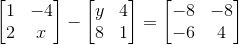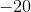Explanation:

When subtracting matrices, you want to subtract each corresponding cell.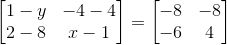Now solve forand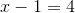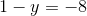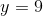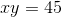### Example Question #1 : Find The Difference Of Two Matrices

If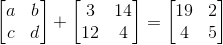, what is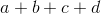?Explanation:

You can treat matrices just like you treat other members of an equation. Therefore, you can subtract the matrix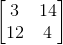from both sides of the equation.  This gives you: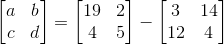Now, matrix subtraction is simple. You merely subtract each element, matching the correlative spaces with each other: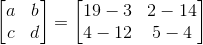Then, you simplify: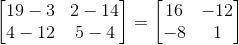Therefore,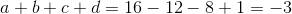### Example Question #1 : How To Subtract Matrices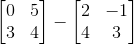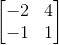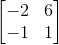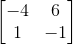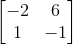Explanation:

When subtracting matrices, subtracting component-wise.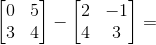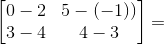### Example Question #2 : How To Subtract Matrices

If, then what is the value of?

0

3

15

12

26

15

Explanation:

Match each term from the first matrix with the corresponding number from the second matrix and subtract.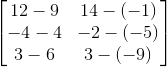Simplify.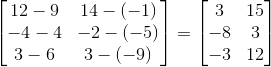Each of the numbers in the matrix solution now corresponds to the letters, a through f. Add a (upper left) and (lower right).### All ACT Math Resources